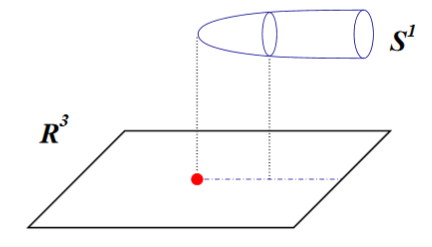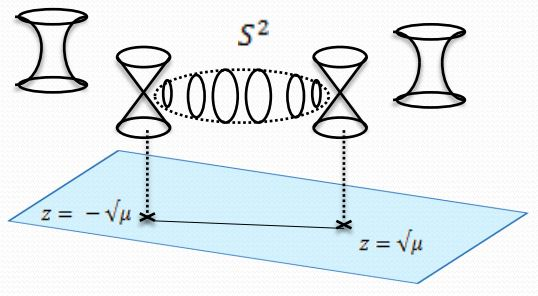# nLab Taub-NUT space

Contents

### Context

#### Riemannian geometry

Riemannian geometry

## Applications

#### Gravity

gravity, supergravity

# Contents

## Idea

A kind of spacetime. For the moment see at KK-monopole for more.graphics grabbed from Acharya-Gukov 04

Consider the left invariant 1-forms on the 3-sphere $\simeq$ SU(2), which in terms of Euler angles are

\begin{aligned} \sigma_1 &= \sin \psi \, d \theta - \cos \psi \sin \theta \, d \phi \\ \sigma_2 & = \cos \psi \, d \theta + \sin \psi \sin \theta \, d \phi \\ \sigma_3 &= d \psi + \cos \theta \, d \phi \end{aligned}

Then the pseudo-Riemannian metric defining the Taub-NUT geometry is

$(d s)^2 = \frac{1}{4} \frac{r+n}{r-n} (d r)^2 + \frac{r-n}{r+n} n^2 {\sigma_3}^2 + \frac{1}{4}(r^2 - n^2)({\sigma_1}^2 + {\sigma_2}^2)$

## Properties

### Relation to KK-monopoles

geometry transverse to KK-monopolesRiemannian metricremarks
Taub-NUT space:
geometry transverse to
$N+1$ distinct KK-monopoles
at $\vec r_i \in \mathbb{R}^3 \;\; i \in \{1, \cdots, N+1\}$
$\array{d s^2_{TaubNUT} \coloneqq U^{-1}(d x^4 + \vec \omega \cdot d \vec r)^2 + U (d \vec r)^2 \,, \\ \vec r \in \mathbb{R}^3,\, x^4 \in \mathbb{R}/(2 \pi R\mathbb{Z}) \\ U \coloneqq 1 + \underoverset{i = 1}{N+1}{\sum} U_i\,, \phantom{AA} \vec \omega \coloneqq \underoverset{i = 1}{N+1}{\sum} \vec \omega_i \\ U_i \coloneqq \frac{R/2}{ {\vert \vec r - \vec r_i\vert} }\,, \phantom{AA} \vec \nabla \times \vec \omega= \vec \nabla U_i}$(e.g. Sen 97b, Sect. 2)
ALE space
Taub-NUT close to $N$ close-by KK-monopoles
e.g. close to $\vec r = 0$: $\frac{{\vert \vec r_i\vert}}{R/2}, \frac{{\vert \vec r\vert}}{R/2} \ll 1$
$\array{d s^2_{ALE} \coloneqq U'^{-1}(d x^4 + \vec \omega \cdot d \vec r)^2 + U' (d \vec r)^2 \,, \\ \vec r \in \mathbb{R}^3,\, x^4 \in \mathbb{R}/(2 \pi R\mathbb{Z}) \\ U' \coloneqq \underoverset{i = 1}{N+1}{\sum} U'_i\,, \phantom{AA} \vec \omega \coloneqq \underoverset{i = 1}{N+1}{\sum} \vec \omega_i \\ U'_i \coloneqq \frac{R/2}{ {\vert \vec r - \vec r_i\vert} }\,, \phantom{AA} \vec \nabla \times \vec \omega= \vec \nabla U_i}$e.g. via Euler angles: $\vec \omega = (N+1)R/2(\cos(\theta)-1) d\psi$
(e.g. Asano 00, Sect. 2)
$A_N$-type ADE singularity:
ALE space in the limit
where all $N+1$ KK-monopoles coincide at $vec r_i = 0$
$\array{d s^2_{A_N Sing} \coloneqq \frac{\vert\vec r\vert }{(N+1)R/2}(d x^4 + \vec \omega \cdot d \vec r)^2 + \frac{ (N+1)R/2}{\vert \vec r\vert} (d \vec r)^2 \,, \\ \vec r \in \mathbb{R}^3,\, x^4 \in \mathbb{R}/(2 \pi R\mathbb{Z}) }$(e.g. Asano 00, Sect. 3)

### Relation to D6-branes

from M-branes to F-branes: superstrings, D-branes and NS5-branes

M-theory on $S^1_A \times S^1_B$-elliptic fibrationKK-compactification on $S^1_A$type IIA string theoryT-dual KK-compactification on $S^1_B$type IIB string theorygeometrize the axio-dilatonF-theory on elliptically fibered-K3 fibrationduality between F-theory and heterotic string theoryheterotic string theory on elliptic fibration
M2-brane wrapping $S_A^1$double dimensional reduction $\mapsto$type IIA superstring$\mapsto$type IIB superstring$\mapsto$$\mapsto$heterotic superstring
M2-brane wrapping $S_B^1$$\mapsto$D2-brane$\mapsto$D1-brane$\mapsto$
M2-brane wrapping $p$ times around $S_A^1$ and $q$ times around $S_B^1$$\mapsto$$p$ strings and $q$ D2-branes$\mapsto$(p,q)-string$\mapsto$
M5-brane wrapping $S_A^1$double dimensional reduction $\mapsto$D4-brane$\mapsto$D5-brane$\mapsto$
M5-brane wrapping $S_B^1$$\mapsto$NS5-brane$\mapsto$NS5-brane$\mapsto$$\mapsto$NS5-brane
M5-brane wrapping $p$ times around $S_A^1$ and $q$ times around $S_B^1$$\mapsto$$p$ D4-brane and $q$ NS5-branes$\mapsto$(p,q)5-brane$\mapsto$
M5-brane wrapping $S_A^1 \times S_B^1$$\mapsto$$\mapsto$D3-brane$\mapsto$
KK-monopole/A-type ADE singularity (degeneration locus of $S^1_A$-circle fibration, Sen limit of $S^1_A \times S^1_B$ elliptic fibration)$\mapsto$D6-brane$\mapsto$D7-branes$\mapsto$A-type nodal curve cycle degeneration locus of elliptic fibration(Sen 97, section 2)SU-gauge enhancement
KK-monopole orientifold/D-type ADE singularity$\mapsto$D6-brane with O6-planes$\mapsto$D7-branes with O7-planes$\mapsto$D-type nodal curve cycle degeneration locus of elliptic fibration(Sen 97, section 3)SO-gauge enhancement
exceptional ADE-singularity$\mapsto$$\mapsto$$\mapsto$exceptional ADE-singularity of elliptic fibration$\mapsto$E6-, E7-, E8-gauge enhancement

(e.g. Johnson 97, Blumenhagen 10)

Discussion in the context of M-theory on G2-manifolds and gauge enhancement